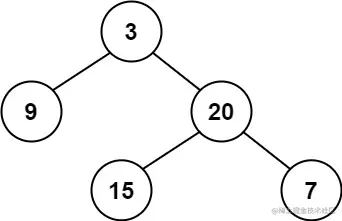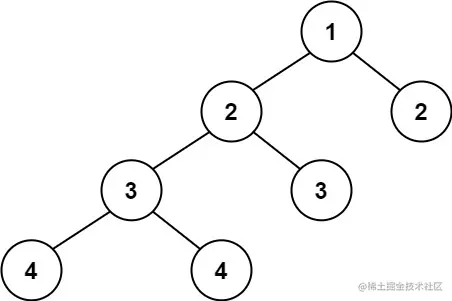# leetcode 110. Balanced Binary Tree（python）

2022-01-30 04:45:36

Little knowledge , Great challenge ！ This article is participating in “ A programmer must have a little knowledge ” Creative activities .

### describe

Given a binary tree, determine if it is height-balanced.

For this problem, a height-balanced binary tree is defined as:

a binary tree in which the left and right subtrees of every node differ in height by no more than 1.

Example 1:``````Input: root = [3,9,20,null,null,15,7]
Output: true
Copy code ``````

Example 2:``````Input: root = [1,2,2,3,3,null,null,4,4]
Output: false
Copy code ``````

Example 3:

``````Input: root = []
Output: true
Copy code ``````

Note:

``````The number of nodes in the tree is in the range [0, 5000].
-104 <= Node.val <= 104
Copy code ``````

### analysis

According to the meaning , A binary tree is given , Check whether the binary tree is highly balanced .

A highly balanced binary tree , It means that the height difference between the left subtree and the right subtree of each node is no more than 1.

This question is actually the same as leetcode 108. Convert Sorted Array to Binary Search Tree The contents of the investigation are the same , It's all about calculating the depth of the tree , I can do that problem, and this problem is similar .

Define a function depth Used to calculate the height of a node , If the node is empty, it will directly return 0 . If it is not empty, continue to drill down into its left and right subtrees to calculate the height of the current node .

Define a function isBalanced It is used to judge whether the current node is a balanced binary tree , adopt depth The height of its subtree can be obtained l and r , Then compare whether the height difference between the left and right subtrees of the current node is less than 2 And the left and right subtrees are also highly balanced binary trees .

``````class TreeNode(object):
def __init__(self, val=0, left=None, right=None):
self.val = val
self.left = left
self.right = right
class Solution(object):
def isBalanced(self, root):
"""
:type root: TreeNode
:rtype: bool
"""
if not root:return True
def depth(root):
if not root:return 0
return max(depth(root.left), depth(root.right)) + 1
l = depth(root.left)
r = depth(root.right)
return abs(l-r)<=1 and self.isBalanced(root.left) and self.isBalanced(root.right)
Copy code ``````

### Running results

``````Runtime: 56 ms, faster than 48.18% of Python online submissions for Balanced Binary Tree.
Memory Usage: 17.6 MB, less than 88.07% of Python online submissions for Balanced Binary Tree.
Copy code ``````